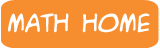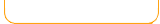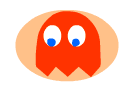Round and Estimate Games

 Decimal GamesKeep checking back for more games!

 RoundEstimateRound Hundreds Estimate Numbers On Number LineRound numbers to the nearest hundred. Estimate numbers by locating them on a number line. Round Thousands Estimate Sums By Rounding Round numbers to the nearest thousand.Estimate sums by rounding each number to the nearest hundred then adding. Round Millions Estimate Differences By Rounding Round numbers to the nearest million. Estimate differences by rounding each number to the nearest hundred then subtracting.Round money amounts to the nearest dollar. Estimate products by rounding the first factor to the nearest ten then multiplying.Round decimals with Scooter Quest.Estimate fractions from the amount of shape colored. Round Mixed Numbers Estimate Integers On a Number LineRound mixed numbers to nearest whole number.Estimate products by rounding the first factor to the nearest ten then multiplying.

 Learn math the fun way with our free educational games! With animation, music, and lots of fun- kids can enjoy practicing math and increase their math skills and math memory. Topics include: addition, subtraction, multiplication, division, fractions, decimals, numbers, geometry, shapes, early math, integers, time, measurement and much more! Game types involve puzzles, action games, arcade style, driving games, swimming, matching, memory and many more varieties for all kinds of learners and abilities. You can also find math videos and math game demonstrations at our math channel on youtube: